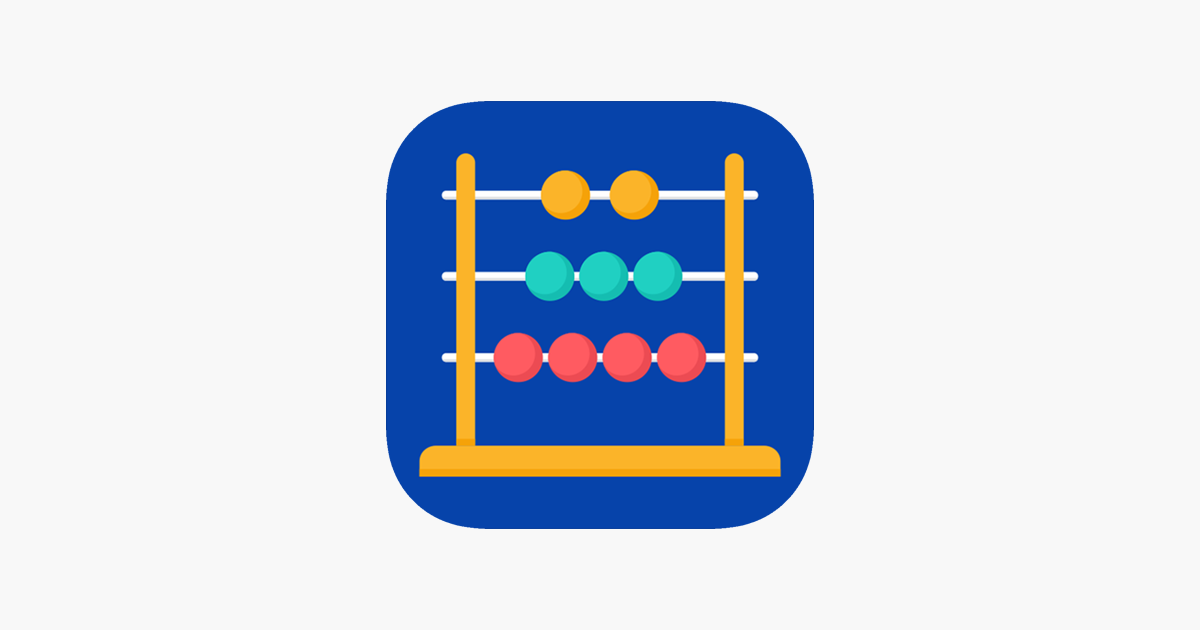# Mental Math Booster – Ranjanben SavaliyaDo you have trouble with basic mental calculations? Sharp your basic maths skills with Mental Math Booster.

Math/Maths Workout is a set of daily brain training exercises and math drills designed to enhance mental arithmetic.

In this math game you will have to show all your knowledge of math and multiplication table to solve arithmetic examples on addition, subtraction, multiplication and division.

Four basic operations,